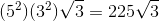## Example Questions

### Example Question #4 : Squaring / Square Roots / Radicals

x2 = 36

Quantity A: x

Quantity B: 6

The relationship cannot be determined from the information given

Quantity B is greater

Quantity A is greater

The two quantities are equal

The relationship cannot be determined from the information given

Explanation:

x2 = 36 -> it is important to remember that this leads to two answers.

x = 6 or x = -6.

If x = 6: A = B.

If x = -6: A < B.

Thus the relationship cannot be determined from the information given.

### Example Question #5 : Squaring / Square Roots / Radicals

According to Heron's Formula, the area of a triangle with side lengths of a, b, and c is given by the following: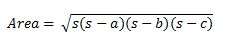where s is one-half of the triangle's perimeter.

What is the area of a triangle with side lengths of 6, 10, and 12 units?

4√14

14√2

8√14

12√5

48√77

8√14

Explanation:

We can use Heron's formula to find the area of the triangle. We can let a = 6, b = 10, and c = 12.

In order to find s, we need to find one half of the perimeter. The perimeter is the sum of the lengths of the sides of the triangle.

Perimeter = a + b + c = 6 + 10 + 12 = 28

In order to find s, we must multiply the perimeter by one-half, which would give us (1/2)(28), or 14.

Now that we have a, b, c, and s, we can calculate the area using Heron's formula.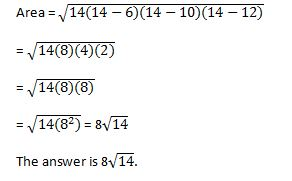### Example Question #1 : New Sat Math No Calculator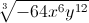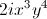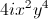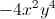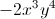Explanation:Look for perfect cubes within each term. This will allow us to factor out of the radical.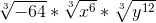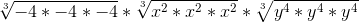Simplify.### Example Question #3 : Squaring / Square Roots / Radicals

Simplify the expression.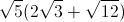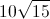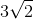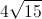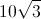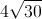Explanation:

Use the distributive property for radicals.Multiply all terms by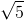.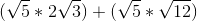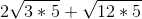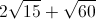Look for perfect square factors under each radical.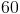has a perfect square of. Thecan be factored out.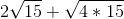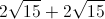### Example Question #8 : Squaring / Square Roots / Radicals

Which of the following expression is equal to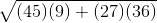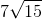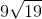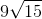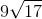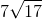Explanation:When simplifying a square root, consider the factors of each of its component parts: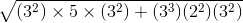Combine like terms: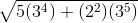Remove the common factor,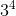: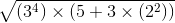Pull the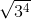outside of the equation as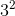: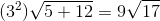### Example Question #9 : Squaring / Square Roots / Radicals

Which of the following is equal to the following expression?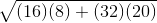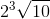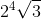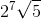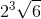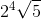Explanation:First, break down the components of the square root: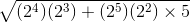Combine like terms. Remember, when multiplying exponents, add them together: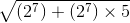Factor out the common factor of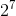: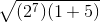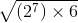Factor the: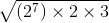Combine the factoredwith the: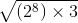Now, you can pull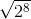out from underneath the square root sign as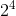:### Example Question #10 : Squaring / Square Roots / Radicals

Which of the following expressions is equal to the following expression?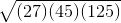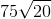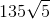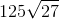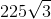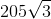Explanation:First, break down the component parts of the square root: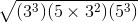Combine like terms in a way that will let you pull some of them out from underneath the square root symbol: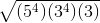Pull out the terms with even exponents and simplify: